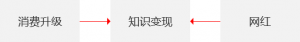## 运营之光

1、装修这个行业虽然发展历程并不算太长，但是市场的空间足够的大，但是大量的传统装修公司仍然依靠着电话营销这样极低效率的方式来获客，这是很有问题的，生存空间必然会受到极大的挤压，那么在这种情况下，就必须有新的方式来帮助更有效率的完成获客这件事情。
2、到今天在电商在很多新兴的互联网产品已经出现了很多的更好的方式，但这些方式其实还并没有出现在装修公司中，包括号称现在的互联网装修公司，大多数也是打着幌子，其实根本就还用着传统的方式，只是说这些新的方式需要时间应用到实践中，不断尝试迭代出来一条适合这个行业的路径出来。
3、这个行业里的人大多数其实还是在思维上的传统，我的意思是僵化的，很难指望这些传统的人能够马上醒悟来意识到需要调整自己来应对未来的竞争，这本身就给了类似我们这样的新兴互联网人很大的空间来去完成这样的使命。

1、其实就是上面提到的这点，如何在消费用户之前，能够给用户创造出来更多的价值？这个挑战确实还是蛮大的。
2、服务的显性化，怎么让你的服务显性化，让你的用户可以感知？现实的问题是什么呢？不是说装修的设计方案或者说施工队长在施工过程中对工地的管控有多么糟糕，而是用户可能并不能很好的感知到成果。那么，怎么让用户可以明显的感知到服务呢？
3、数据，这也是给非常重要的问题，无论是对内还是对外，都需要通过数据来确认结果，来分析过程，但这个恰恰是装修行业存在的问题，你永远都很难记录到这个行业中数据的真实状况，一方面是整个装修过程中涉及到大量的人跟事跟材料跟资金的流转，另一方面是这个行业人本身的原因，并不具备这样的意识去做这些事情。

## 一点运营的小感悟

1、是否大众都可以低成本拥有的东西？
2、是否大众喜好向往的东西？

## 消费升级、知识变现以及网红papi酱酱，公认的视频网红，在视频娱乐方面有选题策划跟表演的天赋，而一票吃瓜群众早已不满足原来的娱乐消遣模式，他们希望有更新的形式（视频），更近的距离（通过微信）来关注自己偶像的动态。而微信的公众号平台作了这样的连接，papi酱酱通过微信（包括微博）这样的平台获得收入，获得关注，获得知名度乃至获得投资。

## Pyhon and Operation

Python看了一段时间语法之后，感觉上已经可以写点小程序了，但真正动手去写的时候，才发现是这个地方报错，那个地方没有得到自己预期的运行结果，多少有些挫败感。

Python希望达到的目标有这么几个：
1、写写爬虫，然后把爬虫爬的数据进行有价值的分析；
2、在Web方面的实际运用，比如搭建个官网啥的；
3、在微信方面的应用——这是我最近看到的一个库，可以实现一个机器人做很多好玩的事情。

1、聚焦的人群是谁？

2、围绕这群人，提供什么样的内容？

3、内容如何持续的产生？

## python的列表(list)、元组(tuple)、字典(dict)

1）一组有序项目的集合。可变的数据类型【可进行增删改查
2）列表是以方括号“[]”包围的数据集合，不同成员以“,”分隔。
3）列表中可以包含任何数据类型，也可包含另一个列表
4）列表可通过序号访问其中成员

1）list.append() 追加成员，成员数据
2）list.pop() 删除成员,删除第i个成员
3）list.count(x) 计算列表中参数x出现的次数
4）list.remove() 删除列表中的成员，直接删除成员i
5）list.extend(L) 向列表中追加另一个列表L
6）list.reverse() 将列表中成员的顺序颠倒
7）list.index(x) 获得参数x在列表中的位置
8）list.sort() 将列表中的成员排序
9）list.insert() 向列表中插入数据insert(a,b)向列表中插入数据

1）不可变序列
2）元组是以圆括号“()”包围的数据集合，不同成员以“,”分隔
3）与列表不同：元组中数据一旦确立就不能改变
4）通过下标进行访问

1）键值对的集合(map)
2）字典是以大括号“{}”包围的数据集合
3）与列表区别：字典是无序的，在字典中通过键来访问成员。
4）字典是可变的，可以包含任何其他类型

M={k1:v1,k2:v2}，访问 M[k1]将得到v1

1) dic.clear() 清空字典
2) dic.keys() 获得键的列表
3) dic.values() 获得值的列表
4) dic.copy() 复制字典
5) dic.pop(k) 删除键k
6) dic.get(k) 获得键k的值
7) dic.update() 更新成员，若成员不存在，相当于加入
8) dic.items() 获得由键和值组成的列表
9) dic.popitem() 随机去除dic中键值对，并且返回这个键值对的值
10) dic.setdefault(‘name’,’new’) 如果dic中有name这个键，那么则返回dic中name对应的值，如果没有则返回new
11) dic.update(another_dic) 用一个字典去更形另外一个字典

## 输入三个整数x,y,z，请把这三个数由小到大输出

```# -*- coding: utf-8 -*-

#输入三个整数x,y,z，请把这三个数由小到大输出。

def inputNumber():
while True:
try:
x = input('input number please:')
#if isinstance(x,(int,float)):
break
except NameError:
print 'Error,input number please:'
return x

def comNumber(x,y,z):
if xy:
print 'from small to big:',y,z,x
else:
print 'from small to big:',y,x,z

x = inputNumber()
y = inputNumber()
z = inputNumber()
print 'your input number is:',x,y,z

comNumber(x,y,z)
```

1、第一次了解了try,except在程序中的应用，实际上一开始没有把要判断异常的语句放在try模块中，导致总是无法执行出预期的结果。
2、comNumber部分的函数应该是凭自己感觉敲的代码，感觉是很挫，没有查资料。等这一轮基础过后再回头看有什么更好的实现方法。

## 输入某年某月某日，判断这一天是这一年的第几天

# -*- coding: utf-8 -*-

#输入某年某月某日，判断这一天是这一年的第几天？

import time,datetime

def input_date():

leapYear = False

while True:
year = raw_input('input year(yyyy):')
if len(year) == 4 and year.isdigit() and year > 0:
if (int(year) % 400 == 0) or (int(year) % 100 != 0 and int(year) % 4 == 0):
leapYear = True
print 'It is leap year'
else:
print 'It is not leap year'
print "your input year is:",year
break
else:
print 'your input is error,input year(yyyy) please!'
#4位，数字，大于o

while True:
month = input('input month(mm):')
if 0<month<13:
print "your input month is:",month
break
else:
print 'your input is error,input year(0<mm<13)again please!'

while True:
date = input('input date(dd):')
if month in [1,3,5,7,8,10,12]:
if 1 <= date <= 31:
bigMonth = True
#print ('your input dates is:%s,%s,%s' % (year, month, date))
break
else:
print 'your input is error,input date(1<=dd<=31) again please!'
elif month in [4,6,9,11]:
if 1<= date <= 30:
#print ('your input dates is:%s,%s,%s' % (year, month, date))
break
else:
print 'your input is error,input date(1<=dd<=30) again please!'
elif month == 2:
if leapYear:
if 1 <= date <= 29:
#print ('your input dates is:%s,%s,%s' % (year, month, date))
break
else:
print 'your input is error,input date(1<=dd<=29) again please!'
else:
if 1 <= date <= 28:
#print ('your input dates is:%s,%s,%s' % (year,month,date))
break
else:
print 'your input is error,input date(1<=dd<=28) again please!'

print ('your input dates is:%s/%s/%s' % (year, month, date))

if month == 1:
numDay = date
elif month == 2:
numDay = 31 + date
elif month == 3:
if leapYear:
numDay = 31 + 29 + date
else:
numDay = 31 + 28 + date
elif month == 4:
if leapYear:
numDay = 31 + 29 + 31 + date
else:
numDay = 31 + 28 + 31 + date
elif month == 5:
if leapYear:
numDay = 31 + 29 + 31 + 30 + date
else:
numDay = 31 + 28 + 31 + 30 + date
elif month == 6:
if leapYear:
numDay = 31 + 29 + 31 + 30 + 31 + date
else:
numDay = 31 + 28 + 31 + 30 + 31 + date
elif month == 7:
if leapYear:
numDay = 31 + 29 + 31 + 30 + 31 + 30 + date
else:
numDay = 31 + 28 + 31 + 30 + 31 + 30 + date
elif month == 8:
if leapYear:
numDay = 31 + 29 + 31 + 30 + 31 + 30 + 31 + date
else:
numDay = 31 + 28 + 31 + 30 + 31 + 30 + 31 + date
elif month == 9:
if leapYear:
numDay = 31 + 29 + 31 + 30 + 31 + 30 + 31 + 31 + date
else:
numDay = 31 + 28 + 31 + 30 + 31 + 30 + 31 + 31 + date
elif month == 10:
if leapYear:
numDay = 31 + 29 + 31 + 30 + 31 + 30 + 31 + 31 + 30 + date
else:
numDay = 31 + 28 + 31 + 30 + 31 + 30 + 31 + 31 + 30 + date
elif month == 11:
if leapYear:
numDay = 31 + 29 + 31 + 30 + 31 + 30 + 31 + 31 + 30 + 31 + date
else:
numDay = 31 + 28 + 31 + 30 + 31 + 30 + 31 + 31 + 30 + 31 + date
elif month == 12:
if leapYear:
numDay = 31 + 29 + 31 + 30 + 31 + 30 + 31 + 31 + 30 + 31 + 30 + date
else:
numDay = 31 + 28 + 31 + 30 + 31 + 30 + 31 + 31 + 30 + 31 + 30 + date

print 'It is No.%s day' % numDay
#def calculate_date():

input_date()

1、无论是年、月、日在输入过程中都判断是否合法，否则重新输入;
3、判断闰年;
2、在计算第几天的时候目前的实现应该还是很挫….

## Python若干函数用法

• sqrt()函数返回值的类型是float
• raw_input()函数返回值类型是string
• input()函数返回值类型是数值，如int、float